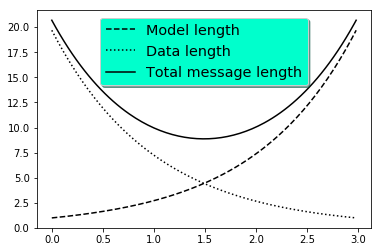## Sunday, December 10, 2017

### Testing: Embedding iPython Notebook on Blogger

In :
import numpy as np
import matplotlib.pyplot as plt

# Make some fake data.
a = b = np.arange(0, 3, .02)
c = np.exp(a)
d = c[::-1]

# Create plots with pre-defined labels.
fig, ax = plt.subplots()
ax.plot(a, c, 'k--', label='Model length')
ax.plot(a, d, 'k:', label='Data length')
ax.plot(a, c + d, 'k', label='Total message length')

legend = ax.legend(loc='upper center', shadow=True, fontsize='x-large')

# Put a nicer background color on the legend.
legend.get_frame().set_facecolor('#00FFCC')

plt.show()## Wednesday, October 18, 2017

### 內積空間

1. $\bracket{\alpha +\beta}{\gamma} = \bracket{\alpha}{\gamma} + \bracket{\beta}{\gamma}.$
2. $\bracket{a\alpha}{\beta} = a\bracket{\alpha}{\beta}.$
3. $\bracket{\alpha}{\beta} = \bracket{\beta}{\alpha}^*.$ (其中，'*'表示共軛複數。)
4. 若$\ket{\alpha}\ne\ket{0}$，則$\bracket{\alpha}{\alpha} > 0$。

$\ket{\alpha} = \sum_i a_i\ket{i}$
$\ket{\beta} = \sum_j b_j\ket{j}$

$\bracket{\alpha}{\beta} = \sum_i\sum_j a_i^* b_j\bracket{i}{j}$

$\bracket{i}{j} = \delta_{ij} = \left\{\begin{array}{l} 1,\,\,\,\, i=j \\ 0,\,\,\,\, i\ne j\end{array}\right.$

$\bracket{\alpha}{\beta} = \sum_i a_i^*b_j$

$\ket{\alpha}\rightarrow \left[\begin{array}{c} a_1 \\ a_2 \\ \vdots \\ a_n \end{array}\right]$

$\ket{\beta}\rightarrow \left[\begin{array}{c} b_1 \\ b_2 \\ \vdots \\ b_n \end{array}\right]$

$\bracket{\alpha}{\beta} = [a_1^*,\, a_2^*,\ldots, a_n^*]\left[\begin{array}{c} b_1 \\ b_2 \\ \vdots \\ b_n \end{array}\right]$

## 向量空間

### 向量加法

1. 任意兩個向量相加，得到另一個向量 ：
$\ket{\alpha} + \ket{\beta} = \ket{\gamma}$
2. 向量加法具備交換性 (commutative)：
$\ket{\alpha} + \ket{\beta} = \ket{\beta} + \ket{\alpha}$
3. 向量加法具備結合性 (associative)：
$\ket{\alpha} + (\ket{\beta} + \ket{\gamma}) = (\ket{\alpha} + \ket{\beta}) + \ket{\gamma}$
4. 存在一個向量$\ket{0}$，稱為零向量 (zero or null vector)，滿足下列性質：
$\ket{\alpha} + \ket{0} = \ket{\alpha}, \,\,\,\,\forall\ket{\alpha}\in\mathbb{V}$
5. 對$\mathbb{V}$中的每一個向量$\ket{\alpha}$，皆存在一個對應的加法反元數 $\ket{-\alpha}$，使得
$\ket{\alpha} + \ket{-\alpha} = \ket{0}$

### 純量乘法

6. 純量與向量相乘之後，得到另一個向量：
$a\ket{\alpha} = \ket{\gamma}$
7.  純量乘法相對於向量加法，具備分配性 (distributive)：
$a(\ket{\alpha} + \ket{\beta}) = a\ket{\alpha} + a\ket{\beta}$
8. 純量加法對純量乘法具備分配性：
$(a+b)\ket{\alpha} = a\ket{\alpha}+b\ket{\alpha}$
9. 一般的乘法與純量乘法具備結合性：
$a(b\ket{\alpha}) = (ab)\ket{\alpha}$
10. 在$\mathbb{F}$中存在一個元素$1$，使得
$1\ket{\alpha} = \ket{\alpha}$

## 線性組合、線性相依與線性獨立

$a\ket{\alpha} + b\ket{\beta} +c\ket{\gamma} + \cdots$

$a\ket{\alpha} + b\ket{\beta} +c\ket{\gamma} + \cdots = \ket{0}$

$\ket{\alpha} = a_1\ket{e_1} + a_2\ket{e_2} + \cdots + a_n\ket{e_n}$

$(a_1, a_2,..., a_n) = \left[\begin{array}{c} a_1 \\ a_2 \\ \vdots \\ a_n \end{array}\right]$

Problem：試證明座標向量為唯一。

$\ket{\alpha} = a_1\ket{e_1} + a_2\ket{e_2} + \cdots + a_n\ket{e_n},$

$\ket{\alpha} = b_1\ket{e_1} + b_2\ket{e_2} + \cdots + b_n\ket{e_n}.$

$\ket{0} = (a_1-b_1)\ket{e_1} + (a_2 - b_2)\ket{e_2} + \cdots + (a_n - b_n)\ket{e_n}$

$\ket{e_j} = -\frac{(a_1 - b_1)}{(a_j - b_j)}\ket{e_1} - \frac{(a_2 - b_2)}{(a_j - b_j)}\ket{e_2} - \cdots - \frac{(a_n - b_n)}{(a_j - b_j)}\ket{e_n}$

## Saturday, October 14, 2017

### 薛丁格方程式

$i\hbar\frac{\partial\Psi}{\partial t} = -\frac{\hbar^2}{2m}\frac{\partial^2\Psi}{\partial x^2} + V\Psi$

$\int^b_a\left|\Psi (x, t)\right|^2 dx = \mbox{時間為t，在位置a與b之間發現粒子的機率}$

## Monday, October 09, 2017

### 機率公設

[A1] 對任意的事件$A$, $0\le P(A)\le 1$。
[A2] $P(S)=1$。
[A3] 若$A_1, A_2, A_3,...$是一個序列的互斥事件 (亦即$A_i\cap A_j =\emptyset$ , $\forall i\ne j$)，則
$P(A_1\cup A_2\cup \cdots) = P(A_1) + P(A_2) + P(A_2) +\cdots$

$P(A) = P(A\cup\emptyset) = P(A) + P(\emptyset)$

$1 = P(S) = P(A\cup A^c) = P(A)+P(A^c)$

$P(B) = P(A)+P(B\setminus A)$

$P(A\setminus B) = P(A) - P(A\cap B)$

$A = (A\setminus B)\cup (A\cap B)$

$P(A) = P(A\setminus B) + P(A\cap B)$

$P(A\cup B) = P(A) + P(B) - P(A\cap B)$

$\begin{array}{rcl} P(A\cup B) & = & P(A\setminus B) + P(B) \\ & = & P(A) - P(A\cap B) + P(B) \\ & = & P(A) + P(B) - P(A\cap B) \end{array}$

## Sunday, July 26, 2015

### GPS衛星的相對論效應

GPS似乎是目前唯一需要考慮相對論效應的商用系統 (或者至少是第一個)。依據狹義相對論(Special Theory of Relativity)，移動中的物體，時間會變慢，而依據廣義相對論 (General Theory of Relativity)，在高處的物體(重力較小)，時間會變快。GPS衛星的移動速度大約每秒4公里(時間變慢)，距離地表約兩萬多公里(時間變快)，兩者的效應並不會完全抵消。相對論效應對GPS的影響，在下列這篇文章中有詳細的推導與說明。

N. Asby and J. J. Spilker Jr., "Introduction to Relativistic Effects on the Global Positioning System," Chapter 13 in Global Positioning System: Theory and Applications Vol. 1, AIAA, 1996.

R. A. Serway, C. J. Moses, and C. A. Moyer, Modern Physics, 3rd Edition, Thomson Brooks/Cole, 2005.

1. 假設GPS衛星運動是一個正圓形軌道，繞行地球一周的週期是11小時58分，依據牛頓運動定律以及萬有引力定律可知：

$\sum F = ma\Rightarrow \frac{GM_E m}{r^2} = \frac{mv^2}{r} = \frac{m}{r}\left(\frac{2\pi r}{T}\right)^2$

$GM_ET^2 = 4\pi^2r^3\Rightarrow r=2.66\times 10^7 \rm{m}$

2. 第二步驟是計算衛星的速率。由第一步驟得到的軌道半徑，以及軌道週期，可以得到衛星的運動速率$v$，如下：

$v = \frac{2\pi r}{T} = \frac{2\pi(2.66\times 10^{-7})\rm{m}}{43080\rm{s}} = 3.87\times 10^3 \rm{m/s}$

3. 第三步驟是計算由於衛星的移動所造成之時間膨脹因子 (狹義相對論)。每一個GPS衛星上都會承載一個振盪器(其基礎振盪頻率為10.23 MHz，其他訊號的頻率都是這個頻率乘上或者除以一個整數)，用以產生GPS訊號的載波頻率。民用的頻率為1575.42 MHz ( = 10.23 MHz $\times$ 154)，或者更精確一點來說，在衛星的參考座標上，訊號的傳送頻率為1575.42 MHz。當地面的使用者接收到衛星訊號時，由於時間膨脹的影響，訊號的頻率會有些微的改變。時間膨脹因子通常以$\gamma$表示

$\gamma = \frac{1}{\sqrt{1-(v/c)^2}}$

$f_{\rm obs} = \frac{\sqrt{1-(v/c)^2}}{\sqrt{1+(v/c)^2}}f_{\rm source}$

$\Delta f_{\rm special} = (1-\gamma)f_{\rm source}$

$\frac{\Delta f_{\rm special}}{f_{\rm source}} = 1-\gamma \approx -8.34\times 10^{-11}$

4. 第四步驟是計算廣義相對論的影響。由於廣義相對論所造成頻率之fractional change，可以表示如下：

$\frac{\Delta f_{\rm general}}{f_{\rm source}} = \frac{\Delta U_g}{mc^2}$

$\Delta U_g = -\frac{GM_Em}{r} + \frac{GM_Em}{\rho}$

$\frac{\Delta f_{\rm general}}{f_{\rm source}} = \frac{\Delta U_g}{mc^2} = +5.29\times 10^{-10}$

$-8.34\times 10^{-11} + 5.29\times 10^{-10} = +4.46\times 10^{-10}$

GPS, Relativity, and Nuclear Detection

## Sunday, January 25, 2015

### Exercises

1. 對任意的$n\times n$矩陣，皆可以找到$n$個特徵值。(True or False)

2. 求下列$\mathbb{R}^3$空間基底之對偶基底(dual basis)：(a) {(1, 0, 0), (0, 1, 0), (0, 0, 1)} (b) {(1, -2, 3), (1, -1, 1), (2, -4, 7)}.

3. 令$\phi$表示$\mathbf{R}^2$的線性泛函(linear functional)，定義為$\phi(x, y) = x - 2y$。若$T$為下列在$\mathbf{R}^2$上的線性映射，試求$(T^t(\phi))(x, y)$，其中$T^t$表示transpose map(有些書寫為$T^*$)：(a)$T(x, y) = (x, 0)$, (b)$T(x, y) = (y, x+y)$, (c) $T(x, y) = (2x-3y, 5x+2y)$。

4. 令$W$為$\mathbb{R}^4$的子空間，$W=span\{(1, 2, -3, 4), (1, 3, -2, 6), (1, 4, -1, 8)\}$。求$W$的零化集(annihilator)之一組基底。

5. 若$\mathbf{A}$為實對稱正定矩陣，試證明$\mathbf{A}=\mathbf{P}^t\mathbf{P}$，其中$\mathbf{P}$是一個非奇異矩陣。

6. 求$\mathbf{A}$的奇異值分解，其中
$\mathbf{A} = \left[\begin{array}{rrr} 1 & 2 &3 \\ 4 & -2 & -1 \end{array}\right]$

7. $\mathbf{A}$是一個regular Markov matrix (每一列加起來皆等於1)
$\mathbf{A} = \left[\begin{array}{rrr} 0.4 & 0.2 & 0.2 \\ 0.1 & 0.7 & 0.2 \\ 0.5 & 0.1 &0.6 \end{array}\right]$